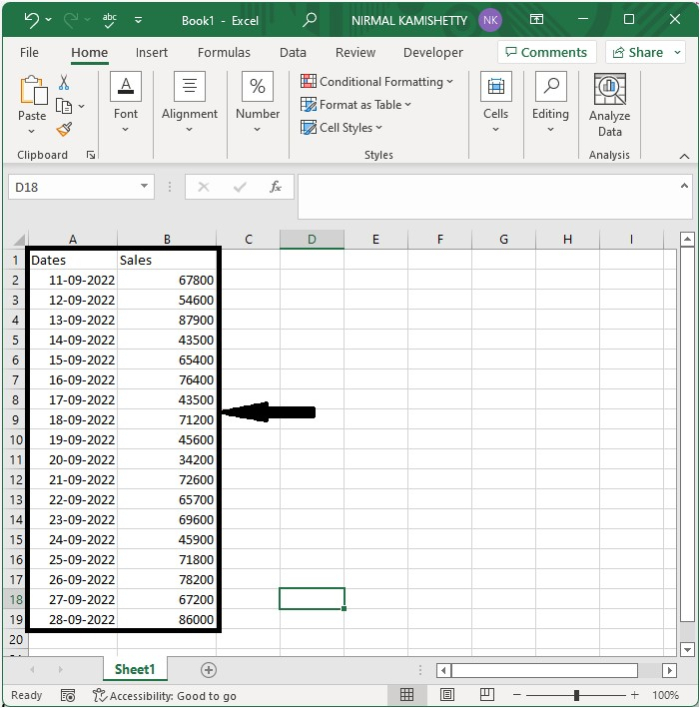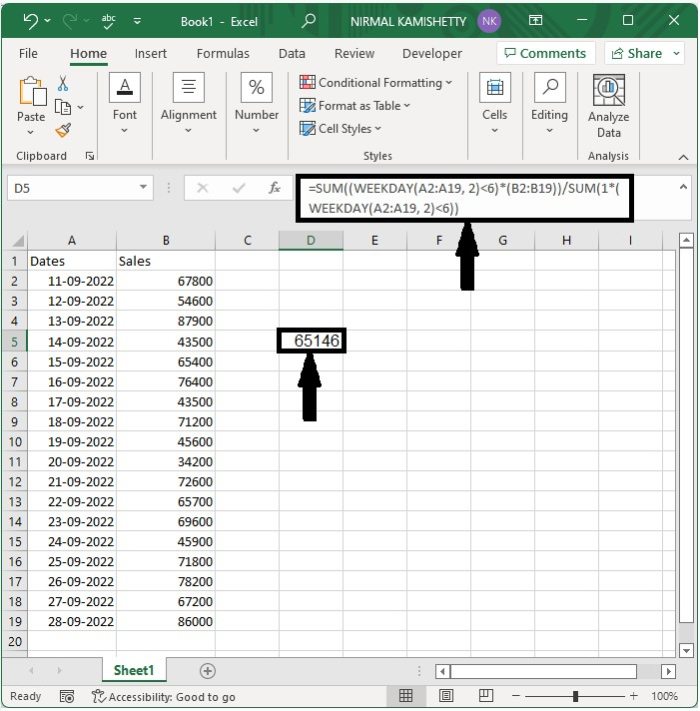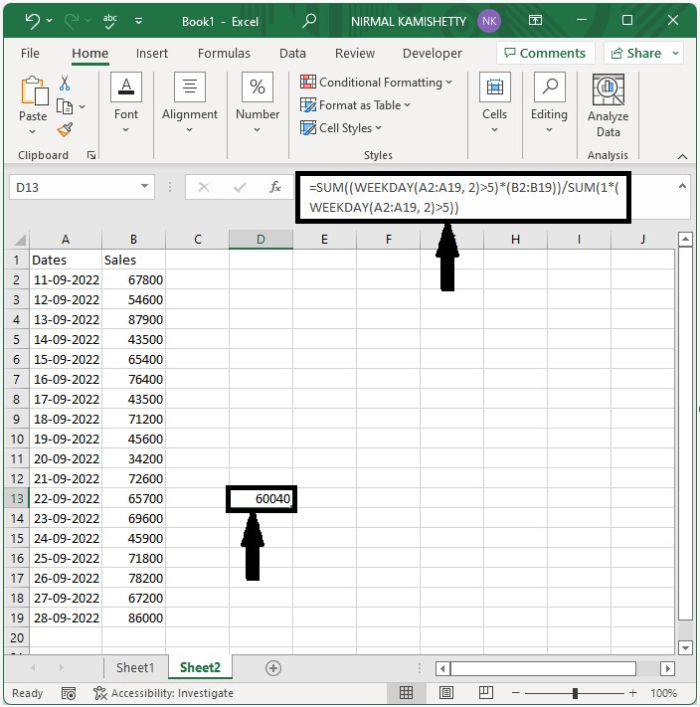# How to Average Data Based on Weekday or Weekend in Excel?

Have you ever tried to find the average of values based on the days of the week? Doing it manually can be an awfully long and time-consuming process. We can do it directly by using the formulas supported by Excel. This tutorial will help you understand how we can average data based on weekdays or weekends in Excel. Here, we have two processes: the first is an average based on weekdays, and the other is based on weekends.

## Average Data Based on Weekday

Here we will use the SUM and WEEKDAY formulas to complete our task. Let us see an effortless process to see how we can average data based on weekdays in Excel.

Let us consider an Excel sheet where the data is like the data shown in the below image.Now click on an empty cell and enter the formula as =SUM((WEEKDAY(A2:A19, 2)<6)*(B2:B19))/SUM(1*(WEEKDAY(A2:A19, 2)<6)) and click Enter to get the values as shown in the below image.

In the formula, A2:A19 is the range of dates, and B2:B19 is the range of values. In the formula, 6 represents the days. We have used "< 6"; the days are Monday, Tuesday, Wednesday, and Thursday.## Average Data Based on Weekends

Here, we will use the SUM and WEEKEND formulas to get the result. Let us see a straightforward process to see how we can average data based on weekends in Excel.

Let us consider the same data that we used in the above example, then click on an empty cell and enter the formula as =SUM((WEEKDAY(A2:A19, 2)>5)*(B2:B19))/SUM(1*(WEEKDAY(A2:A19, 2)>5)) and then click OK to get the result. In the formula, days greater than five are Saturday and Sunday.## Conclusion

In this tutorial, we used a simple example to demonstrate how we can average data based on weekends and weekdays in Excel.

Updated on: 12-Jan-2023

286 Views